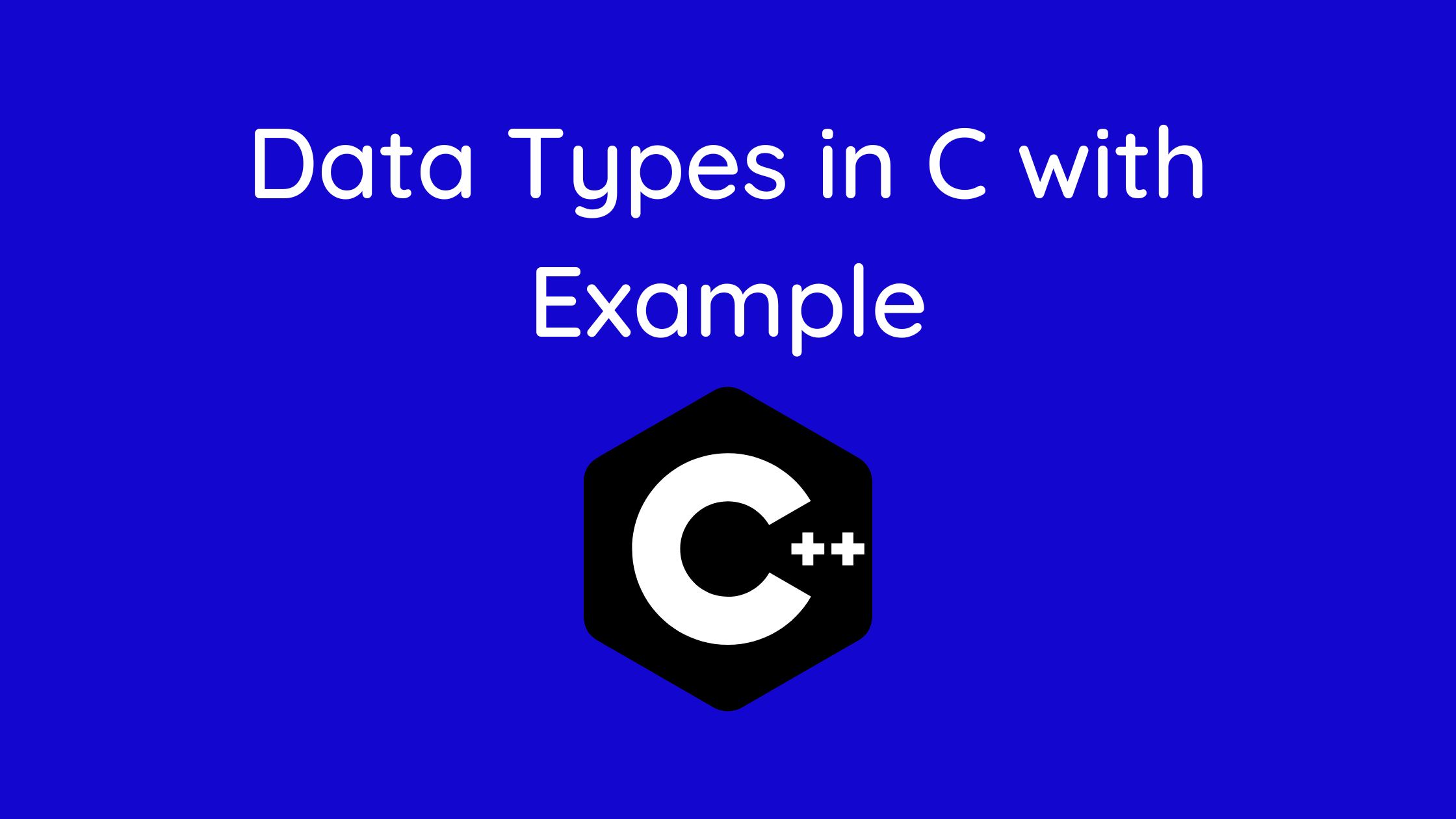## Data Types In C with Example

In this lesson on developers dome, you will learn about fundamental C programming data types like int, float, char, etc.

In C, each variable has a corresponding data type. Each data type has a unique set of operations that can be done on it and varied memory requirements.

Let’s go through each one in brief:

The samples of a few highly popular data types used in C are as follows:

The most fundamental data type in C is char. In practically all compilers, it stores a single character and uses a single byte of memory.

An integer is stored in an int variable, as its name suggests.

Decimal numbers (numbers having floating point values) are stored as single-precision floats.

double: It is used to store double-precision decimal numbers (numbers with floating point values).

Additionally, the ranges that different data types may store numbers in vary. These ranges could change depending on the compiler. The list of 32-bit GCC compiler ranges, together with the memory need and format specifiers, are shown below.

Data types are declarations for variables in C programming. This establishes the kind and volume of data related to variables. For instance,

`int myVar;`

MyVar is an int (integer) type variable in this case. Integers are 4 bytes in size.

Contents

## Simple kinds

Here is a table that provides rapid access to the frequently used types in C programming.

## int

Integers are whole integers that lack decimal values and can have values of zero, one, and both positive and negative signs. For instance, 0, -5, and 10

For declaring an integer variable, we can use int.

`int id;`

Id is an integer-type variable in this context.

In C programming, multiple variables can be declared at once. For instance,

`int id, age;`

Integers typically have a size of 4 bytes (32 bits). And it can exist in any of 232 different states between -2147483648 and 2147483647.

## Data Types: float and double

Real numbers are stored in float and double.

```float salary;
double price;```

Exponential representations of floating-point values are also possible in C. For instance,

`float normalizationFactor = 22.442e2;`

What distinguishes the terms float and double?

Float (a single precision float data type) has a capacity of 4 bytes. Additionally, double is an 8-byte data type that uses double precision.

## Data Types: char

Variables of the character type are declared using the keyword char. For instance,

`char test = 'h';`

The character variable is 1 byte in size.

## Data Types: void

An incomplete type is void. It’s slang for “nothing” or “no kind.” Void can be compared to absence.

For instance, a function’s return type should be void if it returns nothing.

Keep in mind that void-type variables cannot be created.

## Data Types: short and long

Utilize a type specifier long if you need to use a big number. This is how:

```long a;
long long b;
long double c;```

Here, variables a and b both support integer data storage. And C has a floating-point number storage option.

You can use short if you are certain that just a small integer (in the [32,767, +32,767] range) will be used.

`short d;`

Always use the sizeof() operator to determine a variable’s size.

```#include <stdio.h>
int main() {
short a;
long b;
long long c;
long double d;

printf("size of short = %d bytes\n", sizeof(a));
printf("size of long = %d bytes\n", sizeof(b));
printf("size of long long = %d bytes\n", sizeof(c));
printf("size of long double= %d bytes\n", sizeof(d));
return 0;
}```

## Data Types: signed and unsigned

The type modifiers in C are signed and unsigned. Using these, you can change how a data type stores its data:

signed – permits both positive and negative values to be stored.

The ability to store just positive numbers is provided by unsigned.

For instance,

```// valid codes
unsigned int x = 35;
int y = -35;  // signed int
int z = 36;  // signed int

// invalid code: unsigned int cannot hold negative integers
unsigned int num = -35;
```

Because we have used the unsigned modifier in this case, the variables x and num can only store positive and zero values.

Given that int has a capacity of 4 bytes, variable y can store values between -231 and 231-1 while variable x can store values between 0 and 232-1.

## Derived Data Types

Descent from fundamental data types results in derived types of data. Arrays, pointers, function types, structures, etc. are a few examples.

Later lectures will teach us more about these derived data types.

• type bool
• number of types